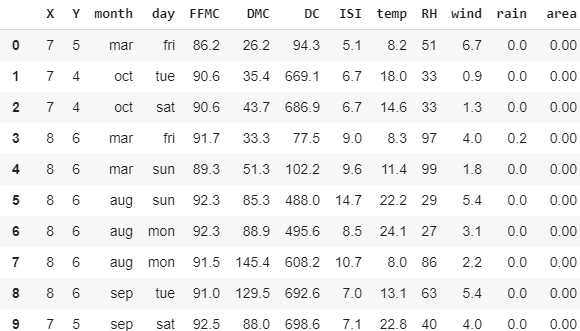# Forest Fire Prediction with the help of multiple regression models to get the best accurate model

Source: Deep Learning on MediumEncoding categorical data

from sklearn.preprocessing import LabelEncoder, OneHotEncoder

labelencoder_X_1 = LabelEncoder()
X[:, 2] = labelencoder_X_1.fit_transform(X[:, 2]) #For month
labelencoder_X_2 = LabelEncoder()
X[:, 3] = labelencoder_X_2.fit_transform(X[:, 3]) #For weekday

onehotencoder = OneHotEncoder(categorical_features = )#dummy variable for month
X = onehotencoder.fit_transform(X).toarray()
X = X[:, 1:]
onehotencoder = OneHotEncoder(categorical_features = )#dummy variable for week
X = onehotencoder.fit_transform(X).toarray()
X = X[:, 1:]

Split data

from sklearn.model_selection import train_test_split
X_train, X_test, y_train, y_test = train_test_split(X, y, test_size = 0.2)

from sklearn.preprocessing import StandardScaler
sc = StandardScaler()
X_train = sc.fit_transform(X_train)
X_test = sc.transform(X_test)

Using Different models

1. Linear Regression:

Linear Regression is a direct way to deal with displaying the connection between a scalar reaction (or dependent variable) and at least one illustrative factors (or independent factors).

from sklearn.linear_model import LinearRegression
model = LinearRegression()
model.fit(X_train, y_train)

from sklearn.metrics import mean_squared_error as mse
from sklearn.metrics import mean_absolute_error as mae
from sklearn.metrics import r2_score

print(‘MSE =’, mse(y_pred, y_test))
print(‘MAE =’, mae(y_pred, y_test))
print(‘R2 Score =’, r2_score(y_pred, y_test))